# Modeling - mutual repressor switch

1. Deterministic model
2. Stochastic model
3. C#Sim model

## Deterministic model of the mutual repressor switch

 Deterministic analysis demonstrated that while theoretical conditions under which the mutual repressor switch would exhibit bistability exist, they are unlikely to occur in a realistic experimental setting. This was because: high transcription factor cooperativity of value 2 or above was required for high-level bistability; even for high cooperativity values, the switch was highly intolerant to even very low leaky gene expression, which is always present to a certain extent in an actual cellular environment. Increase in cooperativity lead to more robust behavior – higher expression levels of stable states were reached and higher – but still low - tolerance to leaky expression was observed. Experimental results confirmed that the mutual repressor switch did not exhibit bistability.

## The model

We can describe the relations for the mutual repressor switch by the following equations. Fractional occupancies of promoters are: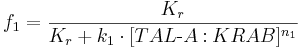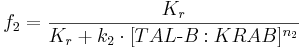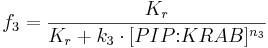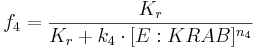where:
• f1, f2, f3 and f4 are probabilities of promoters 1 (construct 1), 2 (construct 2), 3 (construct 3) and 4 (construct 4), respectively, being in an active state, resulting in gene expression;
• [TAL-A:KRAB], [TAL-B:KRAB], [PIP:KRAB] and [E:KRAB] are protein concentrations at a given time;
• k1, k2, k3 and k4 are association constants;
• n1, n2, n3 and n4 are exponents representing the degree of functional cooperativity;
• Kr is the amount of repressor required for 50% repression of constitutive promoter (equal to 1 in our simulations);

ODEs representing protein production are described by a set of equations: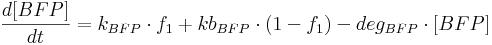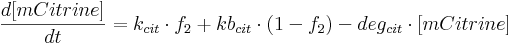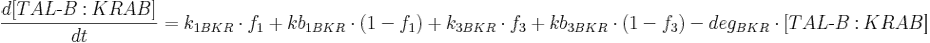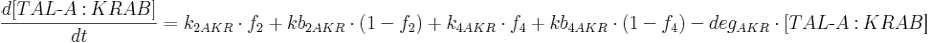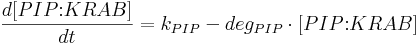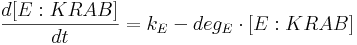where:
• [BFP], [mCitrine], [TAL-A:KRAB], [TAL-B:KRAB], [PIP:KRAB] and [E:KRAB] are protein concentrations;
• kBFP is BFP production rate from construct 1 (i.e. production rate when construct 1 promoter is active);
• kbBFP is basal BFP production rate from construct 1 (i.e. production rate when construct 1 promoter is inactive);
• degBFP is BFP degradation rate;
• kcit is mCitrine production rate from construct 2 (i.e. production rate when construct 2 promoter is active);
• kbcit is basal mCitrine production rate from construct 2 (i.e. production rate when construct 2 promoter in inactive);
• degcit is mCitrine degradation rate;
• k2AKR is TAL-A:KRAB production rate from construct 2;
• kb2AKR is basal TAL-A:KRAB production rate from construct 2;
• k4AKR is TAL-A:KRAB production rate from construct 4;
• kb4AKR is basal TAL-A:KRAB production rate from construct 4;
• degAKR is TAL-A:KRAB degradation rate;
• k1BKR is TAL-B:KRAB production rate from construct 1;
• kb1BKR Is basal TAL-B:KRAB production rate from construct 1;
• k3BKR is TAL-B:KRAB production rate from construct 3;
• kb3BKR is basal TAL-B:KRAB production rate from construct 3;
• degBKR is TAL-B:KRAB degradation rate;
• kPIP is PIP:KRAB production rate;
• degPIP is PIP:KRAB degradation rate;
• kE is E:KRAB production rate;
• degE is E:KRAB degradation rate.

See model derivation for details.

## Simulation results

Simulation results are shown as reporter (BFP, mCitrine) concentrations – indicating one of the two states - as a function of time. No specific units were used, hence no absolute interpretation of the results' values in terms of units is in place. Switching between states was achieved using two signals (signal 1 and signal 2), modeled as a unit step present for a certain duration of time. Each signal was used to induce a different stable state. The presence of signal 1 caused PIP:KRAB to dissociate from promoter 3 binding site and activated transcription. The presence of signal 2 caused E:KRAB to dissociate from promoter 4 binding site and activated transcription.

Initial species' concentrations were 0 for all simulations.

Detailed parameter values for each simulation can be found in MATLAB files here.

### Simulation set 1 - switching between stable states

The purpose of this simulation was to show that conditions exist in deterministic setting where the switch can assume each of the two stable states. Functional cooperativity of each transcription factor (TF) was initialy taken as 2.5. Product production:degradation ratio was equal to 100 (i.e. maximal possible expression level was 100). Ideal circumstances with zero leaky expression were assumed. Figure 1 shows the result of this simulation, demonstrating bistability. The following state-switching scenario was used:

• at time = 0, signal 1 was introduced (signal 1 = 1) to induce the first stable state, depicted by a high level of BFP;
• at time = 100, signal 1 was removed from the system (signal 1 = 0); high-BFP state persisted even after that, meaning a stable state was achieved;
• at time = 400, signal 2 was introduced (signal 2 = 1) to induce the second, high-mCitrine stable state;
• at time = 500, signal 2 was removed from the system (signal 2 = 0); high-mCitrine state persisted, meaning a stable state was achieved;
• at time = 800, signal 1 was re-introduced (signal 1 = 1) to show that a transition to high-BFP state could follow a high-mCitrine state;
• at time = 900, signal 1 was removed (signal 1 = 0); high-BFP stable state persisted.

When low TF exponents values were used – meaning low functional cooperativity - the differentiation between the twoo states steadily degraded after the removal of the inducer and even that only under the conditions when no or very little leaky transcription was present. Figure 2 shows simulation results for the cooperativity value equal to 1.15 for all TFs with zero promotor leakage. Unlike positive feedback loop switch (analyzed in the later chapters), which exhibited high bistability even for cooperativity as low as 1.15, this was not the case for the mutual repressor switch. Increasing E:KRAB and PIP:KRAB production rate in comparison to other proteins did not significantly maintain the stable state levels.

### Simulation set 2 - cooperativity and leaky expression

Higher cooperativity values of TFs were found to increase the robustness of the switch, i.e. higher leaky expression tolerance was achieved, manifesting as higher stable-state levels. Increasing leaky gene expression in the system resulted in the loss of bistability if cooperativity was too low. Bistability was lost regardless of cooperativity after a certain threshold of leaky expression as low as 2% was reached.

Figure 3 shows that introducing leaky expression of each designed repressor caused stable-state levels to drop (to 50 %). Increasing leaky expression further, to 0.3, resulted in extremely low reporter levels with no clear bistability exhibited even in the presence of cooperativity of 2.5, as shown in Figure 4. Increasing PIP:KRAB and E:KRAB production rates relative to other production rates again didn't increase the levels.

### Simulation set 3 - cooperativity and leaky expression

We performed parameter evaluation to find parameter ranges that could lead to bistability. The following state-switching scenario was used for all simulations:

• at time = 0, signal 1 was introduced to induce stable state 1 (high BFP);
• at time = 50, signal 1 was removed;
• at time = 100, signal 2 was introduced to induce stable state 2 (high mCitrine);
• at time = 150, signal 2 was removed.

TF cooperativity was set to 2.5. Product production:degradation ratio was 100. Default leaky expression of all TALs was 0.01, with zero leaky expression of reporters. Simulation result, shown in Figure 5, shows the switch in this scenario exhibited bistability.

We performed several simulations using this scenario, each time varying a different parameter. This way we produced multiple 3D graphs, showing how each of the two stable states changes in time (according to the above-described state-switching scenario) for different parameter values.

First, we tested the effect of TF cooperativity (exponent values) on each state. Figure 6 shows that, for the first (high BFP) stable state, the maximal expression level under given scenario was only observed for cooperativity values above approximately 2. Similarly, as shown in Figure 7, the second (high mCitrine) state reached the maximal level for cooperativity values above 2.

Next, we tested how TAL repressor leaky expression affects state levels. The results – depicted in Figure 8 for state 1 and Figure 9 for state 2- show that, despite TF cooperativity of 2.5, the mutual repressor switch showed very low tolerance to leaky expression, as stability was lost even for values as low as 0.05. Increasing cooperativity improved this tolerance a bit, but insignificantly. Decreasing cooperativity worsened the effect of leaky expression on stability.

Next: Stochastic model of the mutual repressor switch >>